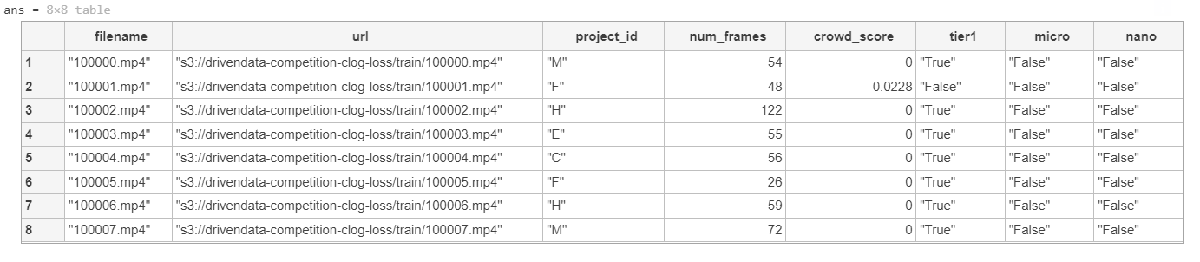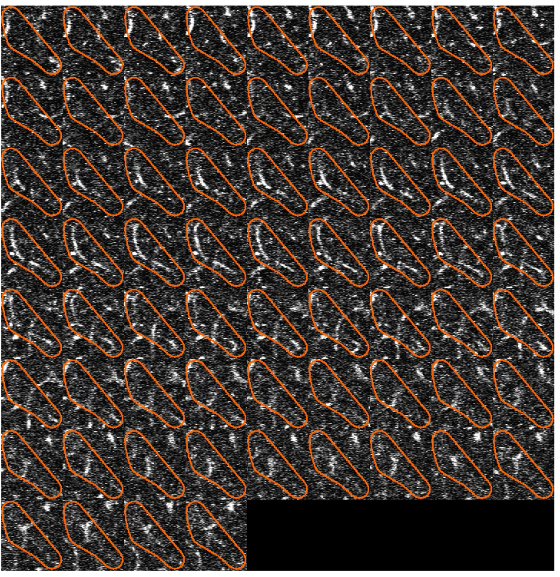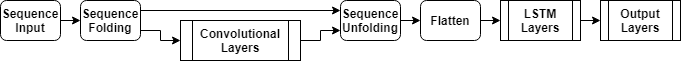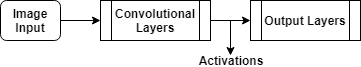# Clog Loss: Advance Alzheimer’s Research with Stall Catchers

## Getting Started with MATLAB

Hello all! We at MathWorks, in collaboration with DrivenData, are excited to bring you this challenge. Through this challenge, you could help put finding an Alzheimer’s treatment target in reach within the next year or two. You will also get the real-world experience of working with a dataset of videos from mice brains. The dataset comes from Stall Catchers, a citizen science effort created by the Human Computation Institute. We encourage you to use MATLAB to train your model by providing complimentary MATLAB licenses. You can also download this MATLAB benchmark code here.
The objective of this challenge is to classify the outlined blood vessel segment as
• flowing if blood is moving through the vessel or
• stalled if the vessel has no blood flow.
The main asset for solving this challenge are the videos themselves! Each video is identified by its filename, which is a numeric string followed by .mp4, e.g., 100000.mp4. All videos are hosted in a public s3 bucket.
The full training dataset contains over 580,000 videos, which is around 1.5 terabytes! To help facilitate faster model prototyping, there are two subset versions of the dataset, referred to as nano and micro.
In addition to the videos, you are provided with "train_metadata.csv" and "test_metadata.csv" files. These files consist of the information like filename, URL of each file, number of frames of each video, nano and micro subsets indication. "train_labels.csv" is the file of labels of training data. You can download these files from competition's Data Download page.
For further details about the dataset check out the Problem Description on the competition webpage.
We are providing a basic Benchmark starter code in MATLAB on the Nanosubset version of the dataset. In this code, we walk through a basic classification model, where we are combining a pre-trained image classification model and an LSTM network. Then, we will use this model to predict the type of the vessel on test data and save a CSV file in the format required for the challenge.
This can serve as basic code where you can start analyzing the data and work towards developing a more efficient, optimized, and accurate model using more of the training data available. Additionally, we have provided a few tips and tricks to work on the complete 1.5TB dataset. On the challenge's Problem Description page, all required details for videos, labels performance and submission metrics are provided.
So, let's get started with this dataset!

To access the variable values from the file train_metadata.csv,load the file in the form of a tabulartext datastore in the workspace.
We can then preview the datastore. This visualizes the first 8 rows of the file.
preview(ttds)You can also import csv files in MATLAB using readtable function. Here, we create training labels from the train_labels.csv file and store it in a form of table. We then convert the values of the variable stalled to categorical, as most deep leaning functions used accept categorical values.
trainlabels.stalled = categorical(trainlabels.stalled);
In this starter code, we will be using the nano subset of the database. Here, we retrieve the files and labels for nano subset from the tables created above and save it in variables nanotrain and nanotrainlabels. (To work with the complete dataset, you will not need this step.)
nanotrain = train(train.nano == 'True',:);
nanotrainlabels = trainlabels(train.nano == 'True',:);

## Acces & Process Video Files

Datastores in MATLAB® are a convenient way of working with and representing collections of data that are too large to fit in memory at one time. It is an object for reading a single file or a collection of files or data. The datastore acts as a repository for data that has the same structure and formatting. To learn more about different datastores, check out the documents below:
In this blog,we used the filedatastore to read each file using its URL. Each file is then processed using the readVideo helper functions, defined at the end of this blog.
We save the datastore in a MAT-file in tempdir or current folder before proceeding to next sections. If the MAT file already exists, then load the datastore from the MAT-file without reassessing them.
tempfds = fullfile(tempdir,"fds_nano.mat");
if exist(tempfds,'file')
else
files = fds.Files;
save(tempfds,"fds");
end
Tip: For working with complete dataset (~1.5TB),create the datastore with the folder location of the training data ('s3://drivendata-competition-clog-loss/train') and not with each url URL to save time and memory. This step can take a long time to run.
(Optional) We can preview the datastore and assure that each video frame is now cropped at the outlined segment.
dataOut = preview(fds);
tile = imtile(dataOut);
imshow(tile);## Classification

To create a deep learning network for video classification:
1. Convert videos to sequences of feature vectors using a pretrained convolutional neural network, such as GoogLeNet, to extract features from each frame.
2. Train an Long Short Term Memory (LSTM) network on the sequences to predict the video labels.
3. Assemble a network that classifies videos directly by combining layers from both networks.
The following diagram illustrates the network architecture.
• To input image sequences to the network, use a sequence input layer.
• To use convolutional layers to extract features, that is, to apply the convolutional operations to each frame of the videos independently, use a sequence folding layer followed by the convolutional layers.
• To restore the sequence structure and reshape the output to vector sequences, use a sequence unfolding layer and a flatten layer.
• To classify the resulting vector sequences, include the LSTM layers followed by the output layers.To convert frames of videos to feature vectors, we use the activations of a pretrained network. Load a pretrained GoogLeNet model using the googlenet function. This function requires the Deep Learning Toolbox™ Model for GoogLeNet Network support package.

### Convert Frames to Feature Vectors

Use the convolutional network as a feature extractor by getting the activations when inputting the video frames to the network.
This diagram illustrates the data flow through the network.The input size should match the input size of the pretrained network, here the GoogLeNet network. The datastore is then resized to the input size using the transform function.
inputSize = netCNN.Layers(1).InputSize(1:2);
fdsReSz = transform(fds,@(x) imresize(x,inputSize));
Convert the videos to sequences of feature vectors, where the feature vectors are the output of the activations function on the last pooling layer of the GoogLeNet network ("pool5-7x7_s1"). To analyze every size and location of the clogged vessels within the outlined segment, we do not modify the lengths of the sequences here.
Tip: After converting the videos to sequences, save the sequences in a MAT-file in the tempdir folder. If the MAT file already exists, then load the sequences from the MAT-file without reconverting them. This step can take a long time to run.
layerName = "pool5-7x7_s1";
tempFile = fullfile(tempdir,"sequences_nano.mat");
if exist(tempFile,'file')
else
numFiles = numel(files);
sequences = cell(numFiles,1);
for i = 1:numFiles
fprintf("Reading file %d of %d...\n", i, numFiles);
'OutputAs','columns','ExecutionEnvironment','auto');
end
save(tempFile,"sequences");
end
We then view the sizes of the first few sequences. Each sequence is a D-by-S array, where D is the number of features (the output size of the pooling layer) and S is the number of frames of the video.
sequences(1:10)
ans = 10×1 cell
1
11024×67 single
21024×59 single
31024×53 single
41024×63 single
51024×66 single
61024×123 single
71024×85 single
81024×54 single
91024×63 single
101024×59 single

### Prepare Training Data

Here, we prepare the data for training by partitioning the data into training and validation partitions. We assign 90% of the data to the training partition and 10% to the validation partition.
labels = nanotrainlabels.stalled;
numObservations = numel(sequences);
idx = randperm(numObservations);
N = floor(0.9 * numObservations);
idxTrain = idx(1:N);
sequencesTrain = sequences(idxTrain);
labelsTrain = labels(idxTrain);
idxValidation = idx(N+1:end);
sequencesValidation = sequences(idxValidation);
labelsValidation = labels(idxValidation);
We then get the sequence lengths of the training data and visualize them in a histogram plot.
numObservationsTrain = numel(sequencesTrain);
sequenceLengths = zeros(1,numObservationsTrain);
for i = 1:numObservationsTrain
sequence = sequencesTrain{i};
sequenceLengths(i) = size(sequence,2);
end
figure
histogram(sequenceLengths)
title("Sequence Lengths")
xlabel("Sequence Length")
ylabel("Frequency")